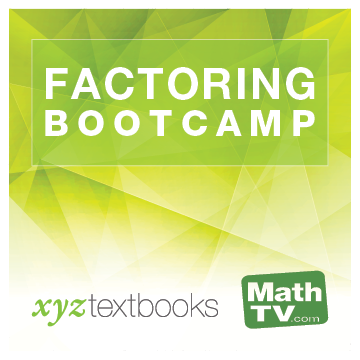#### Videos for:Factoring Bootcamp
Factoring: A General Review

Select an Instructor to Play Video

Mini Lecture
Factor:

1. $$x^2-81$$

2. $$y^2-10y+25$$

3. $$xy-5x+2y-10$$

4. $$3x^2+15xy+18y^2$$

5. $$49x^2+9y^2$$

6. $$6a^2-ab-15b^2$$Innovative products, peer tutors, fair prices, and unlimited access
www.xyztextbooks.com | info@mathtv.com